# GSEB Solutions Class 12 Chemistry Chapter 5 Surface Chemistry

Gujarat Board GSEB Textbook Solutions Class 12 Chemistry Chapter 5 Surface Chemistry Textbook Questions and Answers, Additional Important Questions, Notes Pdf.

## Gujarat Board Textbook Solutions Class 12 Chemistry Chapter 5 Surface Chemistry

### GSEB Class 12 Chemistry Surface Chemistry InText Questions and Answers

Question 1.
Write any two characteristics of chemisorption?

1. Chemisorption is highly specific.
2. Chemisorption involves compound formation and hence is irreversible in nature.Question 2.
Why does physisorption decrease with the increase of temperature?
Physisorption is generally reversible and is exothermic.
Solid + Gas ⇄ Gas/Solid + Heat
Since the adsorption is exothermic, it decreases with increase of temperature.

Question 3.
Why are powdered substances more effective adsorbents than their crystalline forms?
Powdered form has greater surface area. Greater the surface area, greater is the adsorption.

Question 4.
Why is it necessary to remove CO when ammonia is obtained by Haber’s process?
CO acts as catalytic poison and must be removed.Question 5.
Why is the ester hydrolysis slow in the beginning and becomes faster after sometime?
CH3COOC2H5 + H2O → CH3COOH + C2H5OH
In fact, this reaction is catalysed by an acid (H+). Acetic acid formed during the reaction catalyses the hydrolysis of the ester. Therefore, the reaction becomes fast. It is called autocatalyst.

Question 6.
What is the role of desorption in the process of catalysis?
The product formed during catalysis gets detached from the surface of the catalyst by the process of desorption and makes available the catalyst surface for more reaction to occur.Question 7.
Why is it essential to wash the precipitate with water before estimating it quantitatively?
Some amount of impurities remains adsorbed on the surface of the precipitate. It is essential to remove the sticking impurities by washing with water.

### GSEB Class 12 Chemistry Surface Chemistry Text Book Questions and Answers

Question 1.
Distinguish between the meaning of the terms adsorption and absorption. Give one example of each?

 Absorption Adsorption 1. It is the phenomenon in which the particles of gas or liquid get uniformly distributed throughout the body of the solid. 1. It is the phenomenon of of higher concen­tration of particles of gas or liquid on the surface than in the bulk of the solid 2. Concentration is the same throughout the material 2. Concentration on the surface of the adsorbent is higher than that in the bulk 3. Absorption occurs at uniform rate. 3. Adsorption is rapid in the beginning and its rate slowly decreases.

Question 2.
What is the difference between physisorption and chemisorption?

 Physisorption Chemisorption 1. The adsorbate molecules are held on the surface by weak van der Waal’s forces. 1. The adsorbate molecules are held on the surface by chemical bonds. 2. Enthalpy of adsorption is very low (in the range 20-40 kJ mol-1) 2. Enthalpy of adsorption is high (in the range 40-400 kJ mol-1) 3. It is reversible. 3. It is irreversible. 4. It is not very specific in nature. 4. It is highly specific in nature. 5. Forms multimolecular layers. 5. Forms monomolecular layers. 6. It takes place usually at low temperatures and decreases with increase of temperature. 6. It takes place at high temperature. 7. It does not require activation energy. 7. It requires activation energy.Question 3.
Give reason why a finely divided substance is more effective as an adsorbent?
The extent of adsorption increases with the increase of surface area of the adsorbent. The availability of larger surface area in finely divided substances, enable them as effective adsorbents.

Question 4.
What are the factors which influence the adsorption of a gas on a solid?
Factors which affect the adsorption of a gas on a solid are as follows:

1. Nature of gas
2. Surface area of the adsorbent
3. Pressure
4. Temperature

Question 5.
The extent of adsorption of a gas per unit mass of adsorbent ($$\frac{x}{m}$$) depends on the pressure of the gas. A graph drawn between the extent of absorption ($$\frac{x}{m}$$) and the pressure (P) keeping the temperature constant, called adsorption isotherm, is shown in the figure.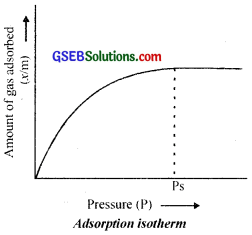Freundlich adsorption isotherm is an equation expressing the relationship between the magnitude of adsorption ($$\frac{x}{m}$$) and pressure (P).
$$\frac{x}{m}$$ = KP1/n where ‘k’ and ‘n’ are constants depending on the nature of adsorbent, adsorbate and temperature.

At low pressure $$\frac{x}{m}$$ ∝ P and at high pressure $$\frac{x}{m}$$ ∝ P i.e., $$\frac{x}{m}$$ is independent of pressure.Question 6.
What do you understand by activation of adsorbent? How is it achieved?
It means increasing the adsorption power of an adsorbent; it is done (i) by increasing the surface area, (ii) by making the surface rough, (iii) by removing the gas already adsorbed.

Question 7.
What role does adsorption play in heterogeneous catalysis?
According to this theory gases are adsorbed by solids forming a unimolecular layer on the surface. Then the adsorbed molecules form a kind of activated complex with the surface atoms. The molecules are adsorbed only at some definite positions known as active centres.

The activated complex formed at these active centres decomposes forming the product. Finally the products get desorbed from the surface. Fresh molecules of the reactants then get adsorbed again and are converted into the products.

For example, the formation of SO3 in contact process due to reaction between SO2 and O2 gases on the surface of Pt catalyst.

The number of active sites on the surface of a catalyst may be increased either by subdivision of the catalyst or by making rough surface on the catalyst. This theory can explain why the catalyst remains unchanged in mass and composition at the end of the reaction. But it fails to explain the action of catalytic promoters and catalytic poisons.

Nature of solid catalysts:
Substances such as metals, alloys of metals, metal oxides, metal suiphides etc. act as solid catalysts. Two important aspects of solid catalysts are discussed below.

(a) Activity:
Activity is the ability of catalysts to accelerate a chemical reaction. A good example of catalytic activity is that of the reaction of H2 and O2 to form H2O in the presence of platinum takes place with an explosive violence. A mixture of pure hydrogen and oxygen does not react at all in the absence of a catalyst.

(b) Selectivity:
It is the ability of a catalyst to direct a reaction to yield a particular product. For example, acetylene on reaction with H2 in the presence of Pt as catalyst gives ethane while in the presence of Lindlar’s catalyst (palladium partially inactivated by quinoline or heavy metal ions) it gives ethylene (ethene).In the presence of different catalysts, H2 and CO give different products.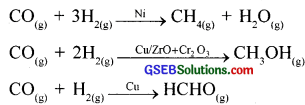Question 8.
Adsorption leads to a decrease in disorder, thus ∆S = +ve. For the process to be performed value of ∆G should be -ve. Thus, in equation ∆G = ∆H – T∆S, ∆G will be negative when ∆H will be negative i.e., exothermic. Thus, adsorption is an exothermic process.

Question 9.
How are the colloidal solutions classified on the basis of physical states of the dispersed phase and dispersion medium?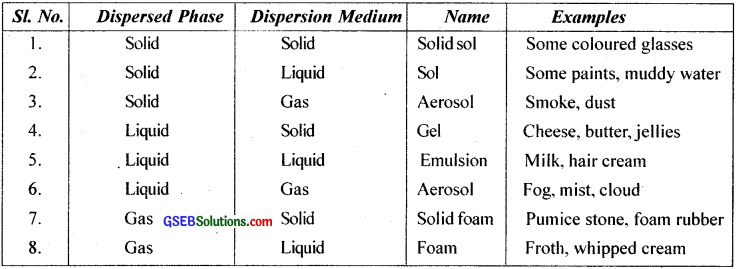Question 10.
Discuss the effect of pressure and temperature on the adsorption of gases on solids?
Adsorption arises due to the unbalanced attractive forces on the surface of the adsorbent. In the bulk of the adsorbent. all the forces acting between the particles are mutually balanced. But on the surface, the particles are not surrounded by atoms or molecules of the same kind. The force of adsorption increases when solid is pulverised or the surface area is increased.

During adsorption, a new bond is fonned between adsorbent and adsorbate. Thus, adsorption is generally exothermic (∆H = -ve). The enthalpy change when one mole of an adsorbate is adsorbed on an adsorbent surface is called heat of adsorption or enthalpy of adsorption. When a gas is adsorbed, the freedom of movement õf its molecules become restricted and hence entropy is decreased (∆S = -ve).

For a process to be spontaneous. ∆G must be negative. On the basis of the equation, ∆G = ∆H – T∆S, ∆G can be negative if ∆H > T∆S. i.e., The process is spontaneous at low temperature. As the adsorption proceeds, ∆H increases and ultimately ∆H becomes equal to T∆S and ∆G becomes zero. At this state equilibrium is attained.

As pressure increases the magnitude of adsorption increases. The variation of adsorption (x/m) with pressure (P) at a constant temperature is shown graphically below. The curve is called adsorption isotherm for the particular temperature. (Here ‘x’ is the amount of gas adsorbed by mass m’ of the adsorbent at particular pressure ‘P’, so that x/m denotes the amount of the gas adsorbed per unit mass of the adsorbent).

At a particular pressure, Ps, x/m reaches a maximum value and then remains constant. This pressure, Ps, is called the saturation pressure or equilibrium pressure. Above this value, pressure has no effect and no more adsorption takes place.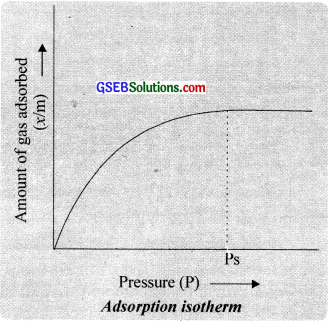The variation of amount of adosorption per unit mass with pressure is expressed by the following empirical equation.
$$\frac{x}{m}$$ = KP1/n ……………… (5.1) where ‘k’ and ‘n’ are constants depending on the nature of the adsorbent, adsorbate and temperature.
Taking logarithms on both sides of the equation we obtain, log $$\frac{x}{m}$$ = log k + $$\frac{1}{n}$$ log P …………………. (5.2)The equations 5.1 and 5.2 are different expressions of the Freundlich adsorption isotherm. Thus a graph between log and log P should give a straight line with an intercept on the log $$\frac{x}{m}$$ axis equal to log k and the slope of the line equal to $$\frac{1}{n}$$.

The factor $$\frac{1}{n}$$ can have values between 0 and 1. At high pressure, $$\frac{1}{n}$$ = 0, ∴ $$\frac{x}{m}$$ = KP0 = k, a constant i.e; the adsorption is independent of pressure.
At low pressure, $$\frac{1}{n}$$ = 1;

∴ $$\frac{x}{m}$$ = KP i.e; ∴$$\frac{x}{m}$$ ∝ P, the adsorption varies directly with pressure. The important drawback of Freundlich adsorption isotherm is that it fails at high pressure.Question 11.
What are lyophilic and lyophobic sols? Give one example of each type. Why are hydrophobic sols easily coagulated?
In lyophilic sols there is a strong interaction between the dispersed phase and the dispersion medium. A layer of the dispersion medium is adsorbed in the dispersed phase and this stabilises the sol, any chemical change which may exist playing a minor role. Lyophilic sols are called reversible sols because if the dispersed phase is separated from the dispersion medium, the sol can be remade by mixing, e.g. Starch, albumin, gelatin etc.

In lyophobic sol there is little or no interaction between the dispersed phase and the dispersion medium. In this type, substances do not pass into the colloidal state by mixing with the dispersion medium. Once the solid particles in lyophobic sol are coagulated or precipitated, they cannot be reconverted into sols by mixing with the dispersion medium. For this reason, lyophobic sols are also called irreversible sols. e.g. Colloidal metals, colloidal hydroxides etc.

Question 12.
What is the difference between multimolecular and macromolecular colloids? Give one example of each. How are associated colloids different from these two types of colloids?
(i) Multimolecular colloids: In this type of colloids the colloidal particles consist of aggregates of atoms or small molecules with a diameter of less than 1 nm. For example, a gold sol may contain particles of different sizes having several atoms of gold. Similarly, sulphur sol contains about one thousand S8 molecules. These are held together by van der Waals’ forces.

(ii) Macromolecular colloids: In macromolecular colloids, the dispersed particles themselves are big molecules, usually polymers. The molecular masses of these macromolecules range from thousands to millions. Synthetic compounds e.g. polyethylene, polystyrene, nylon, rubber, etc. are macromolecules. Since these molecules have dimensions comparable to those of colloidal particles, their dispersions are known as macromolecular colloids. Most lyophillic sols are of this category.

(iii) Associated colloids (Micelles): There are certain substances which behave as normal, strong electrolytes at low concentration, but exhibit colloidal properties at higher concentrations due to the formation of aggregated particles. These are called Micelles. These substances are also called Associated Colloids. Surface active agents like soaps and detergents are of this class.Question 13.
What are enzymes? Write in brief the mechanism of enzyme catalysis?
Enzymes are biological catalysts produced by the living cells which can catalyse the biochemical reaction in living organisms. The mechanism of enzyme action involves the following steps.

(a) Binding of the enzyme to substrate to form a complex
E + S → [ES]

(b) Product formation in the enzyme-substrate complex.
[ES] → EP

(c) Release of products from the enzyme
EP → E + P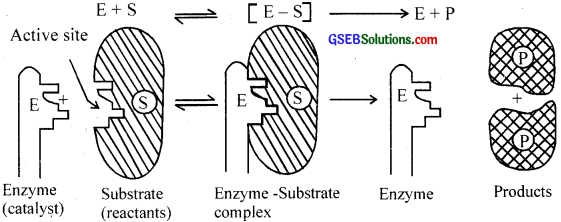(a) The enzyme maltase catalyses the hydrolysis of maltose into glucose.
(b) The enzyme pepsin converts proteins into amino acids.

Question 14.
How are colloids classified on the basis of

1. Physical states of components
2. Nature of dispersion medium and
3. Interaction between dispersed phase and dispersion medium?

The classification of colloids can be done on the basis of

1. The physical states of the dispersed phase and dispersion medium.
2. The interaction or affinity between phases
3. The nature of particles.

Question 15.
Explain what is observed when

1. A beam of light is passed through a colloidal sol.
2. An electrolyte, NaCl is added to hydrated ferric oxide sol.
3. Electric current is passed through a colloidal sol

1. Tyndall effect
2. Coagulation
3. ElectrophoresisQuestion 16.
What are emulsions? What are their different types? Give example of each type?
An emulsion is a colloidal solution of two immiscible liquids in which the liquid acts as the dispersed phase as well as the dispersion medium. These are of two types.

(a) Oil in water (o-in-w) emulsions:
In this type of emulsions, oil acts as dispersed phase and water acts as dispersion medium, e.g. milk

(b) Water in oil (w-in-o) emulsions:
In this type of emulsion, water acts as dispersed phase and oil acts as dispersion medium, e.g. Butter, cod liver oil etc.

Question 17.
What is demulsification? Name two demulsifiers?
The separation of an emulsion into its constituent liquids is called demulsification. This can be done by heating, freezing, centrifuging etc. Dehydrating agents and electrolytes which can destroy the charge on the dispersed phase are effective demulsifiers.

Question 18.
The action of soap is due to emulsification and micelle formation. Comment?
The cleansing action of soap is based on its tendency to form micelles. Dirt sticks to an oily or greasy layer on the fabric. When soap is added to dirty clothes in water, the micelles remain suspended in water entrapping the dirt particles by attaching the hydrophobic end of the soap molecule to the oily layer and the polar end to water. This reduces the interfacial tension between oil and water and the oily dirt particles get displaced in water forming an emulsion. Thus the cloth gets cleaned.

Question 19.
Give four examples of heterogeneous catalysis?
Heterogeneous catalysis:
When the catalyst exists in a different phase from that of reactants, it is said to be a heterogeneous catalyst and the catalysis is called heterogeneous catalysis. Some examples of heterogeneous catalysis are given below.
(i) Manufacture of sulphuric acid by Contact process using V2O5 or Pt as catalyst.(ii) Haber process for the manufacture of ammonia using iron as catalyst.(iii) Oxidation of NH3 into NO in Ostwald’s process using platinum catalyst.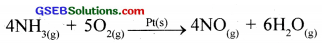(iv) Conversion of vegetable oils to vegetable ghee in presence of Ni catalyst.Heterogeneous catalysts are generally solids and their function is a surface phenomenon. Therefore, heterogeneous catalysis is sometimes called surface catalysis.Question 20.
What do you mean by activity and selectivity of catalysts?
(a) Activity of a catalyst: The activity of a catalyst is its ability to increase the rate of a particular reaction. Chemisorptions are the main factor in deciding the activity of a catalyst. The adsorption of reactants on the catalyst surface should be neither too strong nor too weak. It should just be strong enough to make the catalyst active.

(b) Selectivity of the catalyst: The ability of the catalyst to direct a reaction to yield a particular product is referred to as the selectivity of the catalyst. For example, by using different catalysts, we can get different products for the reaction between H2 and CO.In the presence of differnt catalysts, H2 and CO give different products.Question 21.
Describe some features of catalysis by zeolites?
Zeolites are aluminosilicates having a three-dimensional network structure. Zeolites are highly porous and have cavities of different sizes. The catalytic behaviour of zeolite catalysts depends upon the size of the cavities in them, which usually varies from 260-740 pm. The reactant molecules of a particular shape and size can only enter and get adsorbed. Thus zeolites are called shape-selective catalysts.

Question 22.
What is shape-selective catalysis?
A catalyst reaction is very specific, which depends upon the pore structure of the catalyst and on the size of the reactant and the product. Molecules are called shape-selective catalysis. For example catalyst by zeolite is shape-selective catalysis. The pore size present in the zeolite ranges from 260-740 pm. Molecules having a pore size more than this cannot enter the zeolite and undergo the reaction.

Question 23.
Explain the following terms:

1. Electrophoresis
2. Coagulation
3. Dialysis
4. Tyndall effect.

1. The phenomenon of migration of colloidal particles under the influence of an applied electric field is called electrophoresis. Colloidal particles acquire positive or negative charge due to the formation of Helmholtz double layer. They show electrical properties. The direction of movement can be useful for determining whether a colloidal particle is positively or negatively charged.

2. When sodium chloride is added to a colloidal solution of ferric hydroxide, it gets coagulated. (The process by which a colloidal solution is precipitated by adding a suitable electrolyte is called coagulation or flocculation.)

3. It is a process of removing a dissolved substance from a colloidal solution by means of diffusion through a suitable membrane.

4. When a beam of light is passed through colloidal solution, the path of the beam is illuminated due to Tyndall effect.Question 24.
Give four uses of emulsions?

1. Cleaning action of soaps and detergents are based on emulsification.
2. Emulsions are used as medicines e.g., codliver oil.
3. Many disinfectants like phenyl, dettol, lysol etc., are when mixed with water, form emulsions like oil.
4. Froth floatation process for concentration of ore is based on the principle of emulsification.
5. Digestion of fat by intestine also involves emulsification.

Question 25.
What are micelles? Give an example of a miceller system.
Based on the natute of particle size, colloids are classified as multimolecular colloids, macromolecular colloids and associated colloids.

1. Multimolecular colloids:
In these colloids, the individual particles consist of an aggregate of atoms or small molecules with molecular size less than 1 nm. In these, the particles are held together by van der WaaI’s forces. e.g. Sulphur sol, gold sol, platinum sol etc.

2. Macromolecular colloids:
In these colloids, the particles of dispersed phase are sufficiently big in size to be of colloidal dimensions. e.g. Sols of starch, cellulose, proteins etc. Such colloidal particles have very high molecular mass and are highly stable.

3. Associated colloids:
These are the colloids which behave as normal electrolytes at low concentration but get associated at higher concentration and behave as colloidal solutions. Such colloids are called associated colloids and aggregated particles are called micelles. The formation of micelles takes place only above a particular temperature called Kraft temperature and above a particular concentration called critical micelle concentration (CMC).

On dilution these colloids revert back to individual ions. Micelles may contain as many as 1oo molecules or more.

Mechanism of micelle formation:
Surface active substances like soaps, synthetic detergents etc. are examples of associated colloids. Such substances which form miceller system when dissolved in water, dissociate to give ions.
For example, soap [cg., esodium stearate, CH3(CH2)16COONa) dissociates asThe anion consists oftwo parts- the water dissolving or hydrophilic part (carboxyl group) called head and the water repelling or hydrophobic part (hydrocarbon chain) called the tail. In solution, the hydrophobic chains aggregate by placing the hydrophilic (carboxyl groups) portions near the surface of the micelle, the surface becomes charged. A micelle contains as many as 100 such ions.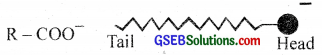Note:
In detergents(eg. CH3 – (CH2)11SO4Na+), the polargroup is – SO4 and the non polar group is the long hydrocarbon chain. Hence the mechanism is same as that of detergents.

Question 26.
Explain the terms with suitable examples:

1. Alcosol
2. Aerosol
3. Hydrosol.

Each of two phases constituting a collodial system may be a gas, a liquid or a solid. The various types of colloidal systems are shown in the table below.Question 27.
Comment on the statement that “colloid is not a substance but a state of substance”?
The given statement is true. It is because colloidal is not the property of a substance. Colloidal is a state in which all the substances can be brought by suitable methods. If the size of the particle lies in the range of 1 nm to 1000 nm, it is in the colloidal state.

### GSEB Class 12 Chemistry Surface Chemistry Additional Important Questions and Answers

Question 1.
What are Lyophilic and Lyophobic sols? Give an example of each type. Which one of these two types of sols is easily coagulated and why? (Delhi 2008)
(i) Lyophilic Sols:
Colloidal sols that are formed by mixing substances such as gum, gelatin, starch etc. with a suitable liquid (dispersion medium) are called lyophilic sols. These sols are reversible in nature i.e. if two constituents of the sol are separated by any means (such as evaporation), then the sol can be prepared again by simply mixing the dispersion medium with the dispersion phase and shaking the mixture.

(ii) Lyophobic sols:
When substances such as metals and their sulphides etc. are mixed with the dispersion medium, they do not form colloidal sols. Their colloidal sols can be prepared only by special methods. Such sols are called lyophobic sols. These sols are irreversible in nature. For example sols of metals.

Now, the stability of hydrophilic sols depends on two things the presence of a charge and the salvation of colloidal particles, on the other hand, the stability of hydrophobic sols is only because of the presence of a charge. Therefore, the latter is very much less stable than the former. If the charge of hydrophobic sols is removed (by addition of electrolytes), then the particles present in them some closer and form aggregate leading to precipitation.Question 2.
Adsorption is found to be a surface phenomenon?

1. What do you mean by physical and chemical adsorption?
2. Explain the term enthalpy of adsorption?
3. List out the important differences between physical adsorption (physisorption) and chemical adsorption (chemisorption)?

1. If the adsorbed molecules are held on the surface of adsorbent by physical forces such as vander Waal’s forces, the adsorption is called physical adsorption and if it is by chemical bonds, is called chemical adsorption.

2. The amount of heat evolved when one mole of an adsorbate is adsorbed on the surface of an adsorbent is called enthalpy of adsorption. Enthalpy of adsorption of physical adsorption is 20-40 kJmoL1 and that of chemical adsorption is 40-400 kJmol-1.

3.

 Physisorption Chemisorption 1. The adsorbate molecules are held on the surface by weak van der Waal’s forces. 1. The adsorbate molecules are held on the surface by chemical bonds. 2. Enthalpy of adsorption is very low (in the range 20-40 kJ mol-1) 2. Enthalpy of adsorption is high (in the range 40-400 kJ mol-1) 3. It is reversible. 3. It is irreversible. 4. It is not very specific in nature. 4. It is highly specific in nature. 5. Forms multimolecular layers. 5. Forms monomolecular layers. 6. It takes place usually at low temperatures and decreases with increase of temperature. 6. It takes place at high temperature. 7. It does not require activation energy. 7. It requires activation energy.

Question 3.
When a small amount of silica gel is placed in a vessel containing moist air, the moisture is adsorbed by silica gel.

1. What do you mean by adsorption?
2. What are the factors which will affect the adsorption of gases on solids?
3. Briefly discuss the influence of pressure on adsorption of a gas on a solid.

1. The phenomenon of attracting and retaining the molecules of a substance on the surface of a liquid or a solid resulting into a higher concentration of the molecules on the surface is called adsorption.

2. Adsorption of gases on a solid depends on

• Nature of gas
• Temperature
• Pressure

3. Increase in pressure at constant temperature increases adsorption. The plots of extent of adsorp¬tion (^-) versus pressure at constant temperature is called adsorption isotherm. The pressure at which reaches a maximum value and then remains constant is called saturation pressure. Above this value, pressure has no effect and no more adsorption takes place.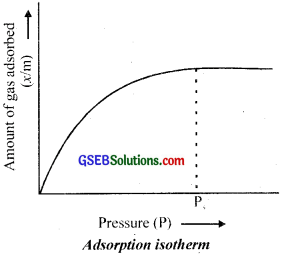Question 4.
A graph showing the adsorption isotherm according to Freundlich isotherm is given.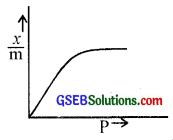(a) How are the extent of adsorption and pressure related?
(b) Draw a plot of log$$\frac{x}{m}$$, to log P and explain how you obtain the value of k and $$\frac{1}{n}$$ .
(a) Extent of adsorption ($$\frac{x}{m}$$) and pressure(p) are related as $$\frac{x}{m}$$ = kP1/n where k and n are constants.
At low pressure, $$\frac{x}{m}$$ = KP
At high pressure, x/m = kP0
At moderate pressure, $$\frac{x}{m}$$ = KP1/n
i. e., log x/m = logk + $$\frac{1}{n}$$ logP

(b)The intercept at log $$\frac{x}{m}$$ axis gives log k, from which k can be obtained.
Slope of the graph gives the value of $$\frac{1}{n}$$Question 7.
It is found that when Litmus is shaken with animal charcoal adsorption takes place
(iv) Explain the term “sorption’.
(ii) The material providing the surface upon which absorption occurs, is known as the adsorbent and the substance adsorbed is called adsorbate.
(iii) The process of removal of an adsorbed substance from the surface of the adsorbent is called desorption.
(iv) The phenomenon in which absorption and adsorption occur together is called sorption.

Question 6.
The teacher asked you to prepare ferric hydroxide sol by adding small amount of FeCl3 to water. But you get a precipitate of Fe(OH)3.

1. Suggest a method to convert Fe(OH)3 precipitate to Fe(OH)3 sol.
2. What is the role of the added substance?
3. What happens when NaCl is added to Fe(OH)3 sol?
4. Give reason.

1. When ferric chloride solution is added to ferric hydroxide percipitate, a colloidal solution of ferric hydroxide is formed.
2. FeCl3 solution acts as the peptizing agent.
3. When sodium chloride is added to a colloidal solution of ferric hydroxide, it gets coagulated. (The process by which a colloidal solution is precipitated by adding a suitable electrolyte is called coagulation or flocculation.)
4. This is because the chloride ions from sodium chloride neutralize the positively charged ferric hydroxide sol.Question 7.
Explain the observations which are likely to be made when

1. A beam of light is allowed to pass through a colloidal solution.
2. An electric current is allowed to pass through a colloidal solution.
3. Explain the chemistry behind each case.